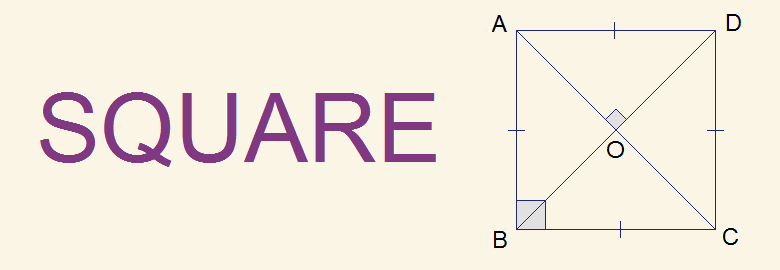# Square | What is Square?## Square | what is Square?

Definition: In geometry, a square is a quadrilateral having its all four sides equal and each angle 90°.
In the given figure of quadrilateral ABCD, AB = BC = CD = AD and A = B = C = D = 90°. So ABCD is a square.

Note:
-   A square is also a parallelogram having all sides equal.
-   A square is also a rectangle having all sides equal.
-   A square is also a rhombus having all angles 90°.

### Length of a square

All four sides of a square are equal and the measure of the side is taken as length (l) of the square. In the given figure of square ABCD,
Length of square = AB = BC = CD = AD = l

### Diagonals of a square

The line joining the opposite vertices of a quadrilateral is called a diagonal. Two diagonals of a square are equal and bisect to each other at 90°.
In the given figure, ABCD is a square. AC and BD are diagonals. They are equal and bisect to each other perpendicularly at point O i.e. AC = BD and AO = CO = BO = DO.

Length of diagonal of a square is given by the formula:

### Perimeter and Area of Square

Perimeter and area of a square are given by the formula:
Perimeter = 4l, where l is the length of side of square.
Area = l2 or ½ d2, where d is the length of diagonal.

### Properties of Square

1.       All sides of square are equal.
2.       Each angle of a square is 90°.
3.       Opposite sides of a square are parallel.
4.       Diagonals of a square are equal.
5.       Diagonals bisect to each other at 90°.

You can comment your questions or problems regarding square here.